# RADIAL VIBRATIONS OF A VISCOELASTIC SPHERICAL SHELL

РАДИАЛЬНЫЕ КОЛЕБАНИЯ ВЯЗКОУПРУГОЙ СФЕРИЧЕСКОЙ ОБОЛОЧКИ
Цитировать:
Almuratov S.N. RADIAL VIBRATIONS OF A VISCOELASTIC SPHERICAL SHELL // Universum: технические науки : электрон. научн. журн. 2022. 3(96). URL: https://7universum.com/ru/tech/archive/item/13224 (дата обращения: 03.06.2023).
Прочитать статью:
DOI - 10.32743/UniTech.2022.96.3.13224

ABSTRACT

Radial vibrations of an elastic isotropic hollow sphere are considered, as well as other limiting cases for the first form of vibrations of a thin spherical shell. The curve dependence of the frequency parameter on the ratio of the thickness of the sphere to its radius is constructed.

АННОТАЦИЯ

Рассмотрено радиальные колебания упругой изотропной пустотелой сферы, также другие предельные случай для первой формы колебаний тонкой сферической оболочки. Построено кривая за­висимость параметра частоты от отношения толщины сферы к ее радиусу.

Keywords: viscosity, elasticity, vibrations, sphere, spherical shell, Poisson's ratio.

Ключевие слова: вязкость, упругость, колебания, сфера, сферический оболочка, коэффициент Пуассона.

Purely radial vibrations of an elastic isotropic hollow sphere is a relatively old problem [1,5]. A more accessible solution is given in [2,4,6], where the frequency equation in the form.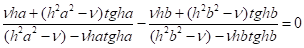(1)

Here a, b are the inner and outer radii of the hollow sphere, respectively.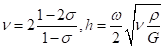(2)

ω-is the complex frequency of radial vibrations, ρ -is the density of the material, σ is the Poisson's ratio, G- is the shear modulus. Frequency calculations are performed only for hollow spheres with negligible stack thickness. The purpose of this report is to derive the frequency equation in a different form, which makes it possible to obtain a very simple solution. By reducing equation (1) to a common denominator and equating the numerator to zero, we obtain(3)

The right side of equation (3) is equivalent to dragged tgh(b-a). Let us now introduce the following notation: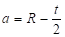,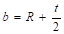,                                                                       (4)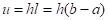,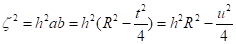Where R- is the radius of the median surface of a thick-walled spherical shell, and t is the wall thickness. We also note that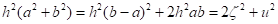Table 1.

Dependence of the fundamental frequency of radial vibrations on the wall thickness

 σ 0 1/3 u hR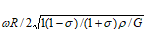t/R hR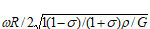t/R 0.00 1.414 1.000 o.ooo 1.414 1.000 0.000 0.25 1.412 0.999 0.177 1.420 1.004 0.176 0.50 1.406 0.994 0.356 1.435 1.015 0.348 0.75 1.394 0.986 0.538 1.460 1.032 0.514 1.00 1.382 0.970 0.729 1.489 1.053 0.671 0.25 1,336 0.945 0.936 1.521 1.076 0.822 1.50 1.279 0.905 1.172 1.551 1.097 0.967 π/2 7.258 0.890 1.248 1.558 1.102 1.008 1,75 1.195 0.845 1.465 1.572 1.112 1.113 2.00 1.076 0.761 1.859 1.577 1.115 1.268 2.082 1,041 0.736 2.000 2.25 1.553 1.098 1.449 2.50 1.483 1.049 1.685 2.744 1.372 1.970 2.000

Now (3) can be rewritten like this: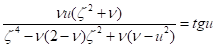(5а)

Or in the form of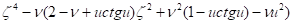(5b)

It is easy to get the solution of equation (5b)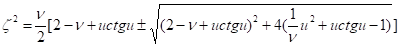(5v)

Since ξ2 should be positive, then only the plus sign should be taken in (5v). Then we get the following frequency equation in parametric form: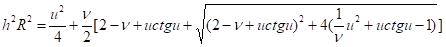(6а)

Using the total equation, it is easy to construct a curve of the dependence of the frequency parameter on the ratio of the thickness of the sphere to its radius. Some results of calculations for the first form of oscillations with several values of the Poisson's ratio (σ = 0, 1/3) are given in Table. 1. These values are given in the form of graphs in Fig. 1 together with the results for a shell made of incompressible isotropic material (σ=0, 1/2) and the results obtained on the basis of the linear theory of shells. It is obvious that the Poisson's ratio of the isotropic shell significantly affects the nature of the change in the fundamental frequency depending on the wall thickness.

We note that the restriction imposed on the value and within the limits when this frequency equation is valid is that and cannot be less than zero (the case of a solid sphere). From equations (4a) and (6b) follows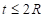(7а)

or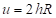(7b)

the equation for bounded values of hR for a solid sphere is now obtained by replacing and by 2hR in the equation (6а):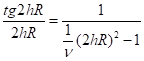(8)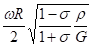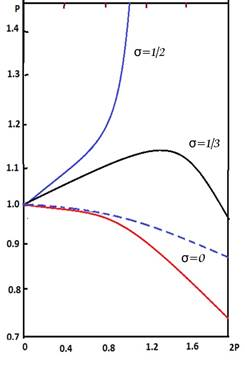Figure 1. Dependence of the frequency of the first mode of oscillation on the ratio of thickness to radius and Poisson's ratio

The same result is obtained by equating the inner radius to zero in equation (3). The solutions are given in  and shown in Table 2.

Таблица 2.

 Root Number σ=0 1/2 1/3 1 0.6620 0.8160 0.8500 0.8733 2 1,8909 1,9285 1.9391 1.9479 3 2.9303 2,9539 2,9606 2.9656 4 3.0485 3.9658 3.9707 3.9744 5 4,9590 4.9728 4.9607 4.9776 6 5.9660 5,9774 5,0806 5.9830

to construct a curve of dependence of the frequency parameter on the ratio of the thickness of the sphere to its radius, we assume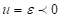(9а)

And from equation (6a) we get then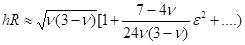(9b)

And from equation (6b)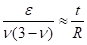(9c)

From here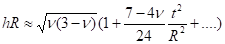(9d)

which gives the dependence of hR on the ratio of thickness x radius. It is interesting to compare this value with the value of n obtained on the basis of linear shell theory. But the expression is easy to find: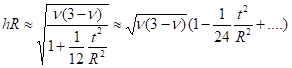(10)

As it is easy to see, the difference between two quantities has an order (t/R)2 in comparison with the unit and is conditioned by the influence of the relative radial vibration of the shell wall, which is taken into account in the shell theory, but is not taken into account in the linear classical theory

We notice from (6b) that at lower frequencies of the thin shell, the thickness t will be small. From equation (6a) we notice that hR tends to infinity or when u is equal to nπ(n≠0). Therefore, for a very thin shell we have

ht=u→nπ                                                              (11)

To find the change in ht for small values of the ratio of thickness to radius, we put

u=nπ+Ɛ                                                             (12a)

Then on (6a) we find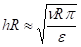(12b)

And from (6a), (12a) and (12b) we get

Ɛ(12b)

from here    (12c)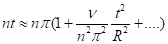(12d)

Finally, we should separately consider the case of an incompressible material when the Poisson's ratio is 1/2. Since with σ tending to 1/2, the magnitude of ν tends to zero, taking into account the expression (2a), we assume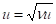(13а)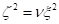(13b)

Where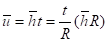(13с)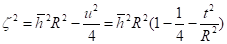(13d)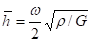(13e)

Then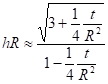(13f)

The values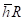depending on t/R are given in the table.3.

Table 3.

Examples of the frequency of the first mode of oscillation for an incompressible material (σ=1/2)

 t/R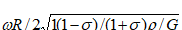0.0 1.7321 1.0000 0.2 1.7525 1.0118 0.4 1.8161 1.0485 0.6 1.9316 1.1152 0.8 2.1162 1.2218 1.0 2.4037 1.3877 1,2 2.8641 1.6535 1.4 3.6629 2.1147 1.6 5.2907 3.0597 1.8 10.273 5.9310 2.0 ∞ ∞

Conclusions.

Based on the results obtained, the following conclusions are made:

1. Purely radial vibrations of an elastic isotropic hollow sphere are considered. The frequency equation is derived in a form that allows us to obtain a very simple solution.

2. The frequency equation is obtained in parametric form and the analysis of the obtained solutions is made. Examples of the frequency of the first mode of oscillations for an incompressible material are carried out.

Literature:

1. Lamb H., “On the Vibrations of a Spherical Shell”, Proceedings of the London Matematical Sociecty, Vol. 14. December 14, 1882, pp. 50-56.
2. Ляв А., «Математическая теория упругости», ОИТИ, 1935.
3. Lamb H., “On the Vibrations of an Elastic Sphero”, Proceedings of the London Matematical Sociecty, Vol. 13, Jung 8 14, 1882, pp. 150-156.
4. Safarov I.I., Almuratov Sh.N., Teshaev M.Kh.,Homidov F.F., Rayimov D.G. Indian Journal of Engineering// On the dynamic stress-strain state of isotropic rectangular plates on an elastic base under vibration loads. 17(47), 2020 . pp. 127-133.
5. N U Kuldashov, Sh N Almuratov, D G Rayimov, F F Homidov and F B Jalolov. Transverse Forced Vibrations of the Plates, the Dissipative Properties of Which are Described Memory Functions. Journal of Physics: Conference Series. 1921 (2021) 012062  doi:10.1088/1742-6596/1921/1/012062.
6. Safarov I.I., N.R,Qulmuratov., M.R.Ishmamatov., N.B.Axmedov., Almuratov Sh.N., ON THE DYNAMIC STRESSED-DEFORMED STATE OF ISOTROPIC RECTANGULAR PLATES ON AN ELASTIC BASE WITH VIBRATION LOADS // International Scientific Journal Theoretical & Applied Science. p-ISSN: 2308-4944 (print) e-ISSN: 2409-0085 (online) Year: 2020 Issue: 02 Volume: 82.
Информация об авторахLecturer at the Tashkent Institute of Chemical Technology. Uzbekistan

преподаватель,  Ташкентского химико-технологического института, Узбекистан, г. Ташкент

Журнал зарегистрирован Федеральной службой по надзору в сфере связи, информационных технологий и массовых коммуникаций (Роскомнадзор), регистрационный номер ЭЛ №ФС77-54434 от 17.06.2013
Учредитель журнала - ООО «МЦНО»
Главный редактор - Ахметов Сайранбек Махсутович.
Top